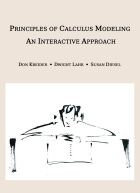# Material Detail## Fundamental Theorem of Calculus: An Investigation

Calculus students are presented with a write-pair-share activity that leads them to a practical understanding of the Fundamental Theorem of Calculus. The activity involves analyzing a function that describes eating speed in a hypothetical dinner table experience. Suitable for either Calculus I or Calculus II students.

Note: This project has a prequel entitled Calculus of the Dinner Table: Mathematical Modeling (listed under Interactive...

Rate

#### Browse...

##### People who viewed this also viewed
••••##### Other materials like Fundamental Theorem of Calculus: An Investigation
••••